## Pearson Type III Distribution

A skewed distribution which is similar to the Binomial Distribution when(Abramowitz and Stegun 1972, p. 930).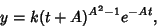(1)

forwhere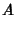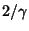(2)(3)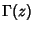is the Gamma Function, andis a standardized variate. Another form is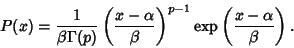(4)

For this distribution, the Characteristic Function is(5)

and the Mean, Variance, Skewness, and Kurtosis are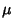(6)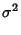(7)(8)(9)

References

Abramowitz, M. and Stegun, C. A. (Eds.). Handbook of Mathematical Functions with Formulas, Graphs, and Mathematical Tables, 9th printing. New York: Dover, 1972.